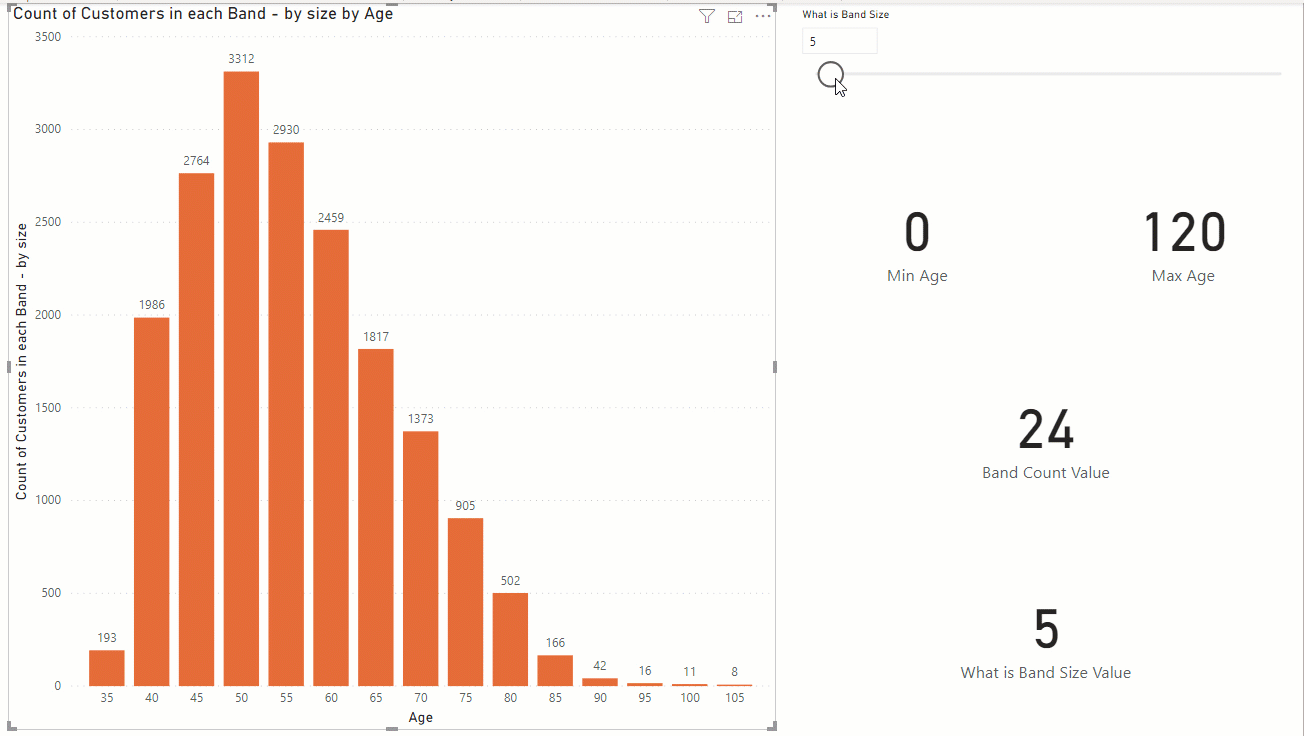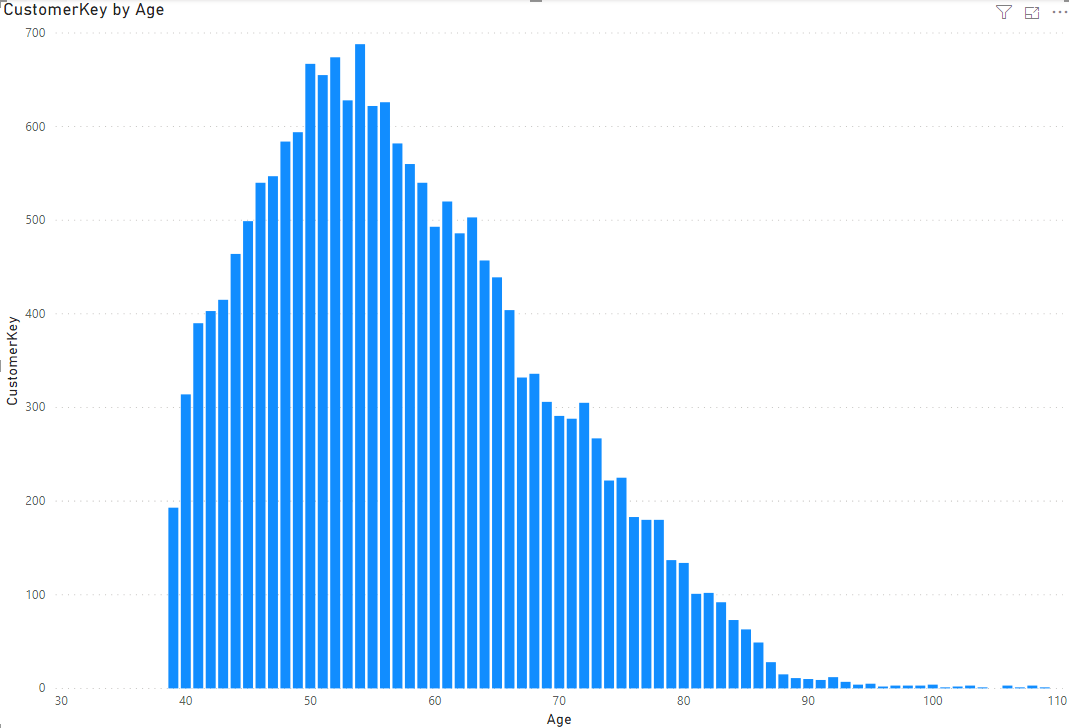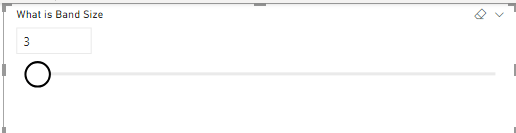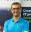# Dynamic Banding or Grouping in Power BI – Using DAX Measures – Choose the Size of BinsIn the previous article/video, I explained how to create dynamic bins by choosing the count of bins in a slicer in the Power BI report. In this article, I’ll explain, how you can do it the other way around, which is by selecting the size of the bin, you will have bins and buckets dynamically generated.

## Banding, Grouping or Binning

Banding (or grouping or binning), means splitting a numeric data type into buckets or groups. Let’s say, we have customers with all ages, but we want to group them in age buckets of 10. one bucket would be 1 to 10, another from 11 to 20, etc. Here is an example of those buckets in the X-Axis of a chart;Banding can be done statically using any of the methods below;

## Sample Dataset

I have a DimCustomer table with a few columns about each customer, and one of the columns is Age. If you are interested to learn how the age is calculated, read my article here about calculating the age in Power Query.## Desired Dynamic Banding

However, sometimes, you need the banding to be dynamic. Dynamic banding means that user can select (through a slicer) how many buckets (or bins or bands) is needed, and then the calculation changes based on that. Here is what we need:I have also explained how you can implement dynamic banding by the count of bins in the previous article, which you can read about it here:

#### Dynamic Banding or Grouping in Power BI – Using DAX Measures – Choose the Count of Bins

Most of the process is similar to the previous article, but because this can be a need on its own, I have added that separately here in full details.

## Age Band Table

This solution is only one of the methods for implementing this scenario. There are, of course, other methods too. In this method, First I have strated with creating an Age Band table with all possible age values.

`Age Band = GENERATESERIES(0,120,1)`This table is then related to the DimCustomer using the Age column;The relationship above means that I can have Age from the Age Band table as the X-Axis to filter the count of customers in the DimCustomer table;Now for the dynamic banding, here is the list of steps:

## Size of Bands; What-If Parameter

Create a What-if parameter for the size of bands,the what-if parameter to start from the minimum size of band you want (let’s say 1), to maximum (let’s say 120), and the increment value (let’s say 1). That would create a table like this:

`What is Band Size = GENERATESERIES(1, 120, 1)`

this is how the table looks like:When you add the what-if parameter, you will also have a measure using the SELECTEDVALUE as below;

`What is Band Size Value = SELECTEDVALUE('What is Band Size'[What is Band Size], 1)`

This what-if parameter is now a slicer on your page.## DAX Measures

You can add a few measures for helping with the final calculation, or combine them all in one measure. I split it here, because it helps the process of learning. Here are my measures:

Min Age is the measure that shows the minimum age value;

`Min Age = MINX(ALL('Age Band'),'Age Band'[Age])`

Max Age is showing the maximum age value;

`Max Age = MAXX(ALL('Age Band'),'Age Band'[Age])`

Band Count Value is a measure that calculates what is the count of the bands, based on the size of the band, and minimum and maximum value; (the count of bands is not needed for the main calculation, it is just added here for extra information);

`Band Count Value = DIVIDE([Max Age]-[Min Age],[What is Band Size Value])`

For example, if the band size (What is Band Size) in the slicer is selected 3, then the count of bands is (120-0)/3=40.### Count of Customers in Each Band

This is the measure that does the main function of our dynamic banding. This measure’s expression first finds out what is the band of the current age in the X-Axis, and calculates the count of customers for that band, but only shows the value for the band’s head (which is the age that the band starts from). Here is the expression:

```Count of Customers in each Band - by size =
var _minAge=[Min Age]
var _maxAge=[Max Age]
var _bandSize=[What is Band Size Value]
var _AgeBandTable=GENERATESERIES(_minAge,_maxAge,_bandSize)
var _currAge=SELECTEDVALUE('Age Band'[Age])
FILTER(
_AgeBandTable,
[Value]<=_currAge),[Value])
return
CALCULATE(
COUNTROWS(DimCustomer),
))```

To explain parts in this expression, here are the details:

_AgeBandTable is a virtual table of all bands, this is created dynamically;

`var _AgeBandTable=GENERATESERIES(_minAge,_maxAge,_bandSize)`

_currAge is giving us the Age value in the X-Axis:

`var _currAge=SELECTEDVALUE('Age Band'[Age])`

The _bandHead calculation is a filter of the _ageBandTable to find out the band for the selected Age;

```var _bandHead=MAXX(
FILTER(
_AgeBandTable,
[Value]<=_currAge),[Value])```

Based on the _bandHead and size, now the Tail is calculated:

`var _bandTail=_bandHead+_bandSize`

The return value is the count of rows from DimCustomer when the age value is between head and tail;

```if(_currAge=_bandHead,
CALCULATE(
COUNTROWS(DimCustomer),
))```

Altogether, this measure returns the count of customers in each age band that is dynamically selected by the slicer;

```Count of Customers in each Band - by size =
var _minAge=[Min Age]
var _maxAge=[Max Age]
var _bandSize=[What is Band Size Value]
var _AgeBandTable=GENERATESERIES(_minAge,_maxAge,_bandSize)
var _currAge=SELECTEDVALUE('Age Band'[Age])
FILTER(
_AgeBandTable,
[Value]<=_currAge),[Value])
return
CALCULATE(
COUNTROWS(DimCustomer),
))```

And you can test the final results here. As you see, the user can dynamically choose how many bands are needed, and everything changes based on that;Until the next DAX article, have fun DAXing!

## Video

Trainer, Consultant, Mentor
Reza Rad is a Microsoft Regional Director, an Author, Trainer, Speaker and Consultant. He has a BSc in Computer engineering; he has more than 20 years’ experience in data analysis, BI, databases, programming, and development mostly on Microsoft technologies. He is a Microsoft Data Platform MVP for 12 continuous years (from 2011 till now) for his dedication in Microsoft BI. Reza is an active blogger and co-founder of RADACAD. Reza is also co-founder and co-organizer of Difinity conference in New Zealand, Power BI Summit, and Data Insight Summit.
Reza is author of more than 14 books on Microsoft Business Intelligence, most of these books are published under Power BI category. Among these are books such as Power BI DAX Simplified, Pro Power BI Architecture, Power BI from Rookie to Rock Star, Power Query books series, Row-Level Security in Power BI and etc.
He is an International Speaker in Microsoft Ignite, Microsoft Business Applications Summit, Data Insight Summit, PASS Summit, SQL Saturday and SQL user groups. And He is a Microsoft Certified Trainer.
Reza’s passion is to help you find the best data solution, he is Data enthusiast.
His articles on different aspects of technologies, especially on MS BI, can be found on his blog: https://radacad.com/blog.

## 2 thoughts on “Dynamic Banding or Grouping in Power BI – Using DAX Measures – Choose the Size of Bins”

•Jose Jones says:

Hi Reza,

Thank you so much for your example! This works great, but in a context of a huge dataset, the performance of this measure is not acceptable. I’ working on huge dataset in direct connectivity with a Data Brick Spark cluster. Is there another avenue you could think of? Thank you very much for your amazing contribution to the power Bi community!

•Reza Rad says:

Hi Jose
For a huge dataset, I would suggest pre-defined banding using Power Query or any other ETL ways.
Cheers
Reza